My name is Steven and I am a tutor/lectuer (Specialised in Math, Science, Accounting and Computing) This site (sgteachers.com) is created by me and has used by many teachers and parents and hope this site could continue to be useful to the educators. For this coming holiday, I am conducting programming courses (1 to 1 at your home or my place - Jurong West St 65) as following: Python Programming (Age 11 - 18) Scratch Programming (Age 7 - 11) I also conduct tuition on: GCE O level Computing (Syllabus 7155) Cambridge IGCSE Computer Science GCE A level (H2) Computing (Syllabus 9569) Cambridge International AS and A Level Computing NCC Diploma in Computer Studies SMS and Whatapps me now to find out more @ 98772403.

 Total subscribers: 6120
Math Worksheets GeneratorSign UpAddition (2 digits)Addition (2 digits) Without Regrouping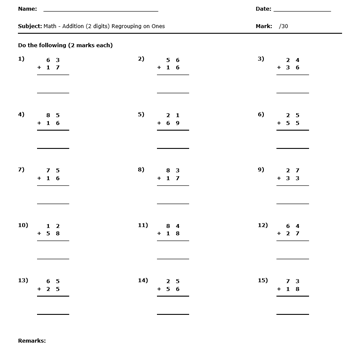Addition (2 digits) With Regrouping On One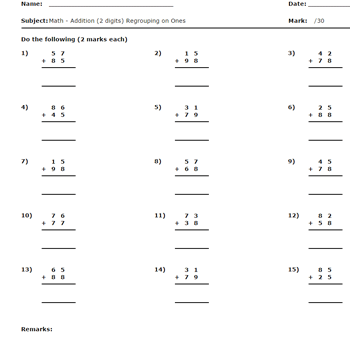Addition (2 digits) With Regrouping On One and TenAddition (3 digits)Subtraction (2 digits)Subtraction (2 digits) With No RegroupingSubtraction (2 digits) With RegroupingSubtraction (3 digits)Multiplication (1 Digit)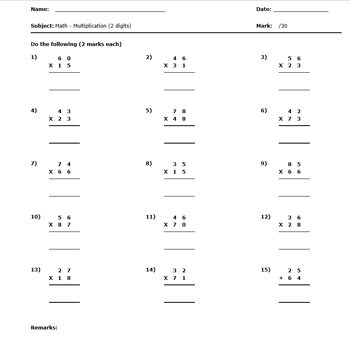Multiplication (2 Digit)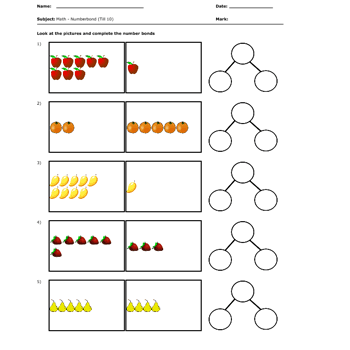Number Bond Till10 Complete using picture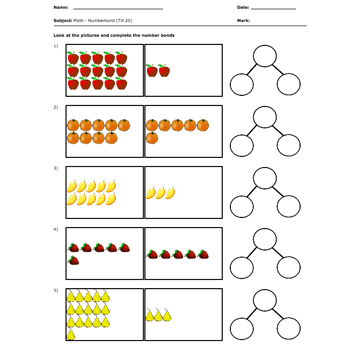Number Bond Till 20 Complete using picture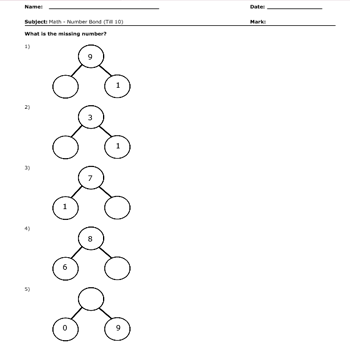Number Bond Till 10 Fill the missing number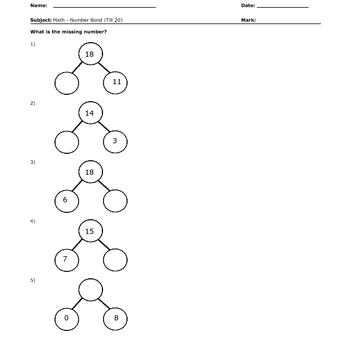Number Bond Till 20 Fill the missing number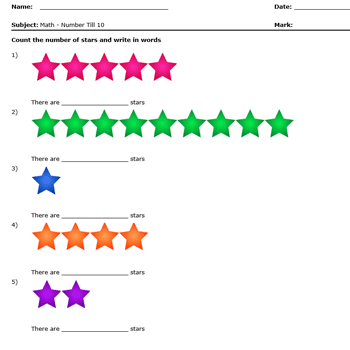Number Till 10 Count and write in words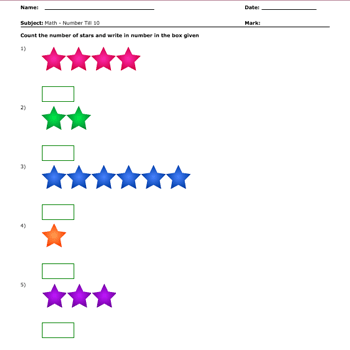Number Till 10 Count and write in numbersNumber Till 100 Count and write in words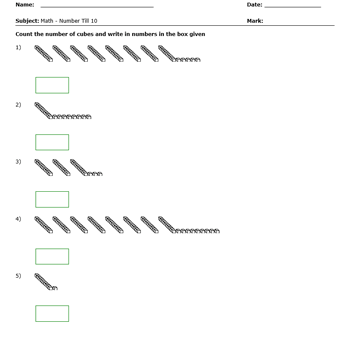Number Till 100 Count and write in NumbersCompare Number Till 10 Comparing by Counting Tick the greater numberCompare Number Till 10 Comparing by Counting Cross the smaller number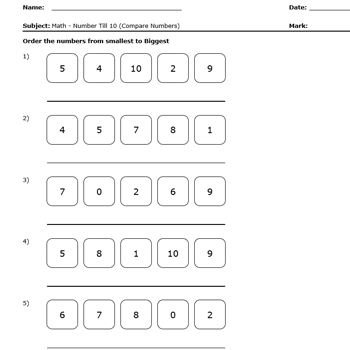Order Number (Upper Limit 10) From Smallest to LargestOrder Number (Upper Limit 10) From Largest to SmallestOrder Number (Upper Limit 100) From Smallest To LargestOrder Number (Upper Limit 100) From Largest to SmallestMatch Number To Words From 1 Till 10Write Number In Words Till 10Convert Number To Words Till 20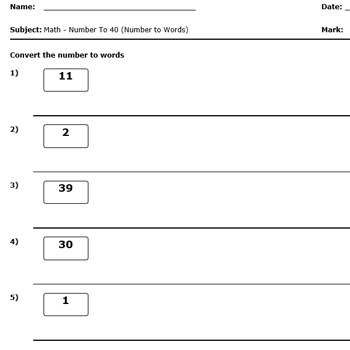Convert Number To Words Till 40Convert Number To Words Till 100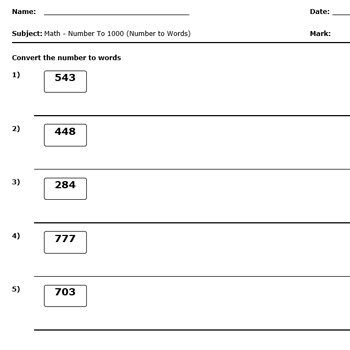Convert Numbers To Words Till 1000Convert Numbers To Words Till 10000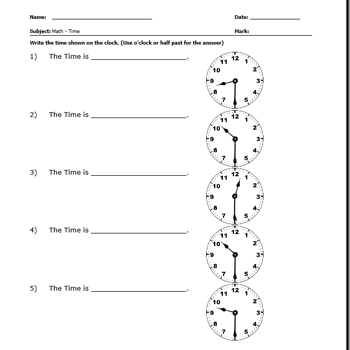Time Tell The TimeTime Determine The TimeFraction Find the numerator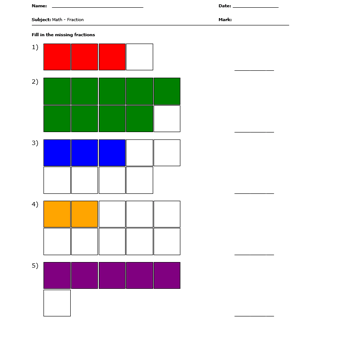Fraction Get the fraction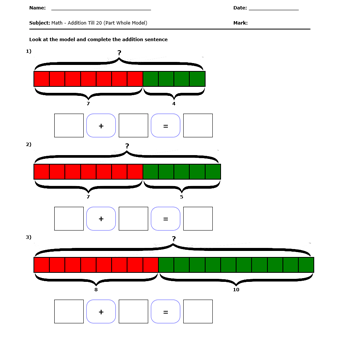Addition (Part Whole Model)Addition (Comparison Model)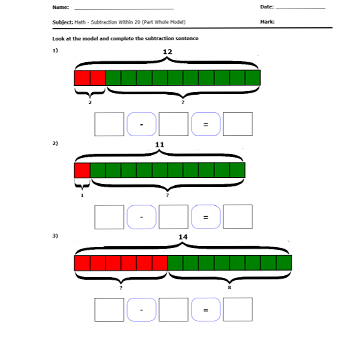Subtraction (Part Whole Model)Subtraction (Comparison Model)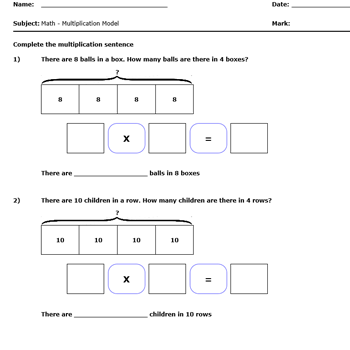Multiplication (Using Model Diagram)Subtraction (Using Model Diagram)Bar Graph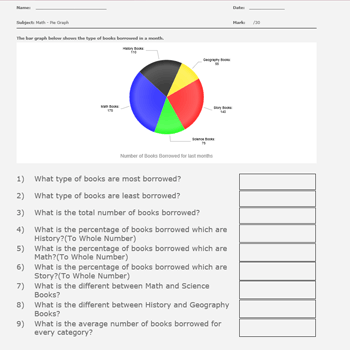Pie Graph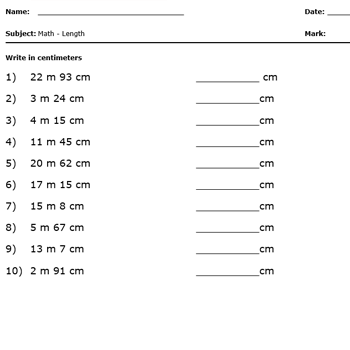Length (Write in Centimeters)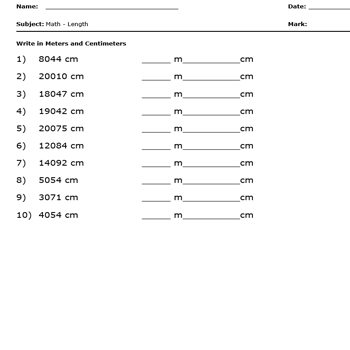Length (Write in Metres and Centimeters)Length (Write in Metres)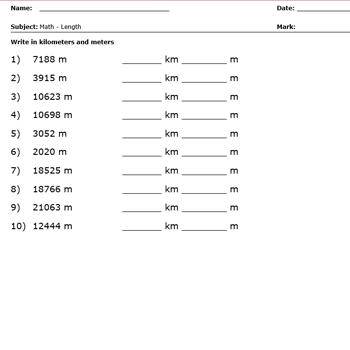Length (Write in Kilometers and Metres)Weight (Write in grams)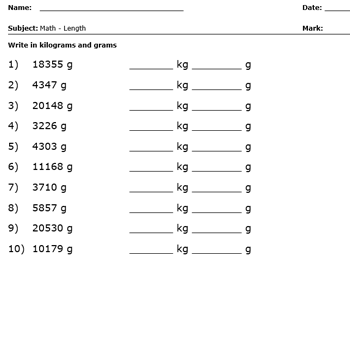Weight (Write in kilograms and grams)Capacity (Write in Millilitres)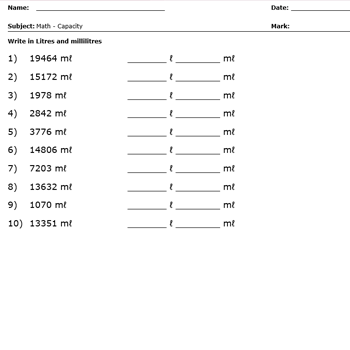Capacity (Write in Litres and Millilitres)